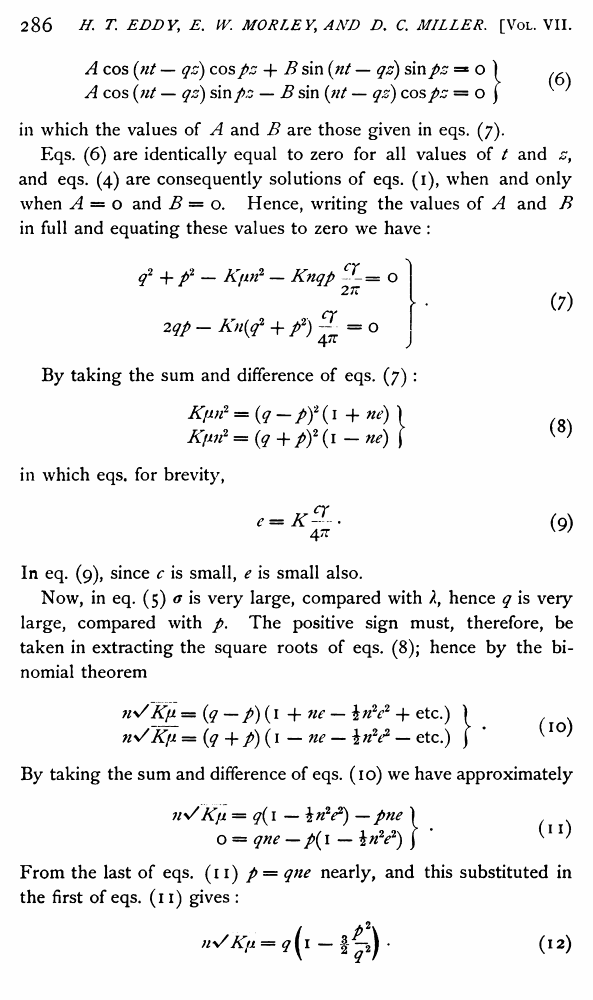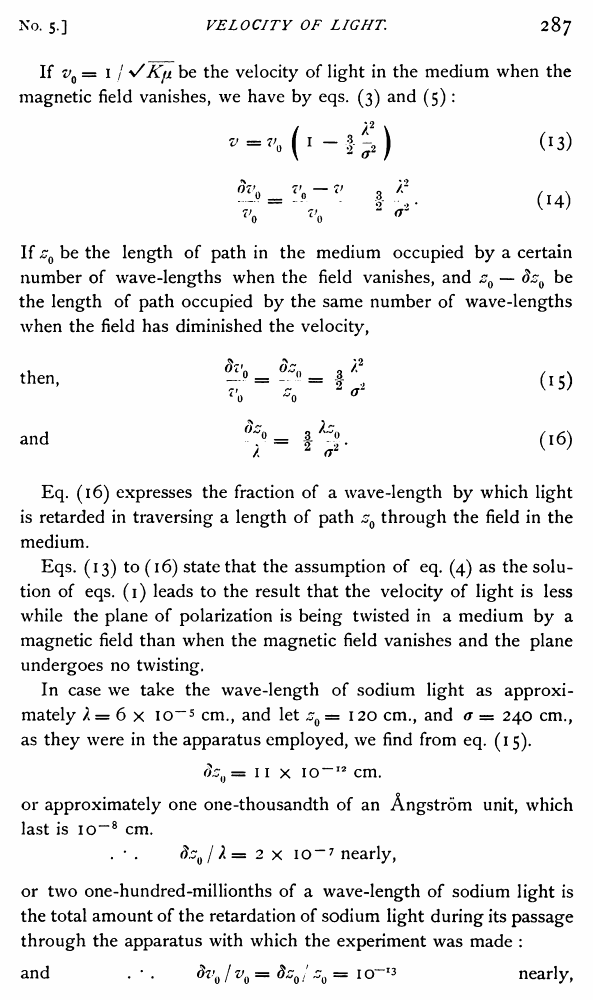Henry T. Eddy, Edward W. Morley, Dayton C. Miller. The Velocity of Light in The Magnetic Field //Physical Review (Series I), Volume 7, Issue 5 (1898)

В начало   Другие форматы   <<<     Страница 286   >>>283  284  285  286 287  288  289  290  291  292  293  294  295  296

A cos {fit — qz) cos pz + B sin {fit — qz) sin pz = o ) ,

A cos {fit — qz) sin pz — B sin {fit — qz) cos pz — of

in which the values of A and B are those given in eqs. (7).

Eqs. (6) are identically equal to zero for all values of t and zy and eqs. (4) are consequently solutions of eqs. (1), when and only when A = o and B = o. Hence, writing the values of A and B in full and equating these values to zero we have :

in which eqs. for brevity,

In eq. (9), since c is small, e is small also.

Now, in eq. (5) a is very large, compared with k, hence q is very large, compared with p. The positive sign must, therefore, be taken in extracting the square roots of eqs. (8); hence by the binomial theorem

ny/~K(JL = (q — /) (1 + ne — in2e2 + etc.) )

n\SKfi = (q -f p) (1 — ne — — etc.) ) * ^I0^

By taking the sum and difference of eqs. (10) we have approximately

nVKfi = q(i — in2e2) — pne )

o = qne — p{\ — \n2e2) j ^1

From the last of eqs. (11) p = qne nearly, and this substituted in the first of eqs. (n) gives :

q2 + f - Kim2 - Knqp O

(7)

By taking the sum and difference of eqs. (7) :

Ki»i2 = {q-pf{ 1 + ne) ) Kim2 = (q + py (1 — ne) j

If v0 = I / VKji be the velocity of light in the medium when the magnetic field vanishes, we have by eqs. (3) and (5):

VmtVo (! -f^2) (13)

t£- (14)

If z0 be the length of path in the medium occupied by a certain number of wave-lengths when the field vanishes, and z0 — dz0 be the length of path occupied by the same number of wave-lengths when the field has diminished the velocity,

then, - 0 = = I- (15)

70 ~0 ~ °

and °"° = (16)

/ z <jz v '

Eq. (16) expresses the fraction of a wave-length by which light is retarded in traversing a length of path s0 through the field in the medium.

Eqs. (13) to (16) state that the assumption of eq. (4) as the solution of eqs. (1) leads to the result that the velocity of light is less while the plane of polarization is being twisted in a medium by a magnetic field than when the magnetic field vanishes and the plane undergoes no twisting.

In case we take the wave-length of sodium light as approximately / = 6 x io-5 cm., and let zQ = 120 cm., and a — 240 cm., as they were in the apparatus employed, we find from eq. (15).

(hi} = 11 x io~12 cm.

or approximately one one-thousandth of an Angstrom unit, which last is io“8 cm.

. • . dz0/ ?,= 2 x io-7 nearly,

or two one-hundred-millionths of a wave-length of sodium light is the total amount of the retardation of sodium light during its passage through the apparatus with which the experiment was made :

and . * . <J?'0 / v0 = dz0/ z0 = io~13 nearly,